Question

# Solve for maturity value, discount period, bank discount, and proceeds.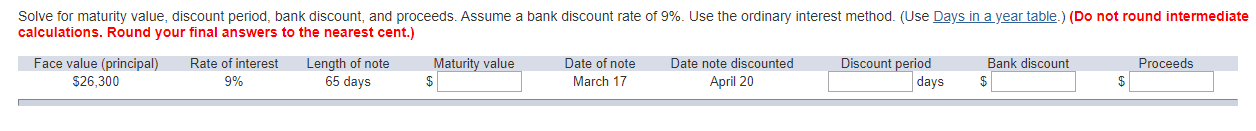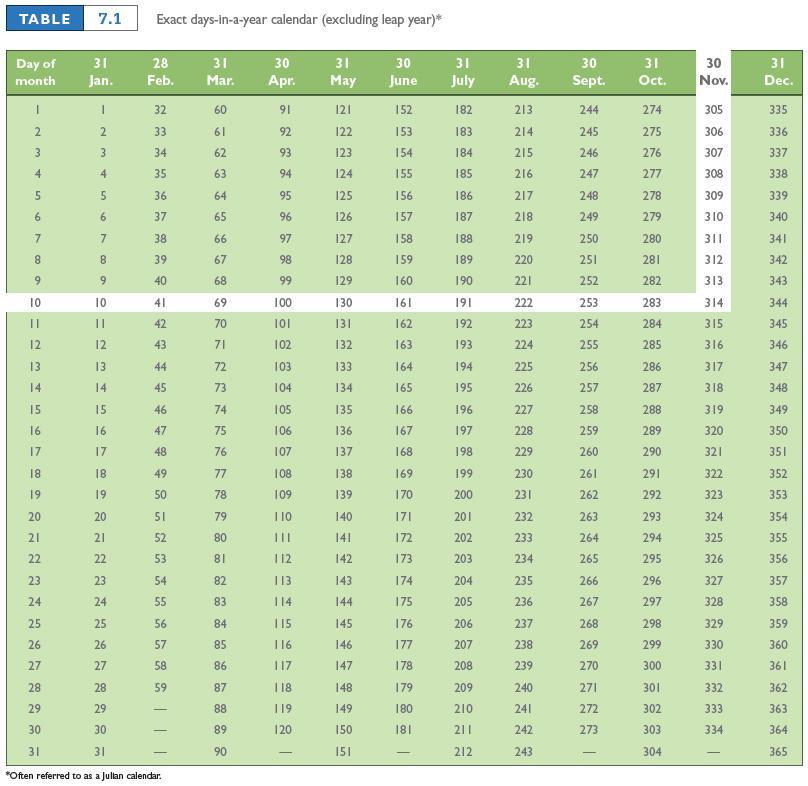Solve for maturity value, discount period, bank discount, and proceeds. Assume a bank discount rate of 9%. Use the ordinary interest method. (Use Days in a year table.) (Do not round intermediate calculations. Round your final answers to the nearest cent.)

Face Value = 26300

bank discount rate or rate of interest = 9%

Length of note = 65 days

So, interest = 26300* 9% * 65/360 = \$427.38

Maturity Value = 26300+ 427.38= \$26727.38

Discount Period = 34-65 = 31 days

Bank Discount = \$26727.38 * 9% * 31/360 = \$207.14

Proceeds = Maturity Value - Bank Discount = \$26727.38 - \$207.14= \$26934.52

Face Value P = \$26300

Bank discount rate D =9%

Rate of interest R = 9%

Length of note LN = 65 days

Since Interest I =PRT

Therefore Interest I = \$26300(9/100)(65/360) = \$427.375=\$427.38

Since Maturity Value MV = P+I

Therefore Maturity Value MV = \$26300+ \$427.38= \$26727.38

Since Discount Period DP = LN - (Day of discount in table - day of note in days table)

Therefore  Discount Period DP = 65 - (110-76) = 65-34 = 31 days

Since, Bank Discount DB = (MV)(D)((DP)

Therefore Bank Discount DB = \$26727.38(9/100)(31/360) = \$207.137195 = \$207.14

Since Proceeds PS = MV - BD

Therefore Proceeds PS = \$26727.38 - \$207.14= \$26520.24

#### Earn Coins

Coins can be redeemed for fabulous gifts.

Similar Homework Help Questions
• ### Bill Blank signed an \$7,540 note at Citizen's Bank. Citizen's charges a 8.2% discount rate. Assume...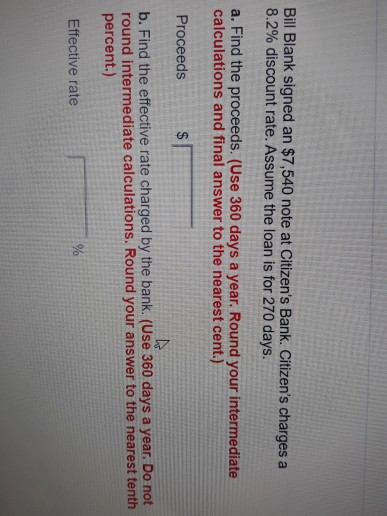Bill Blank signed an \$7,540 note at Citizen's Bank. Citizen's charges a 8.2% discount rate. Assume the loan is for 270 days. a. Find the proceeds. (Use 360 days a year. Round your intermediate calculations and final answer to the nearest cent.) Proceeds b. Find the effective rate charged by the bank. (Use 360 days a year. Do not round intermediate calculations. Round your answer to the nearest tenth percent.) Effective rate You were offered the opportunity to purchase either...

• ### On September 12, Jody Jansen went to Sunshine Bank to borrow \$2,300 at 9% interest. Jody...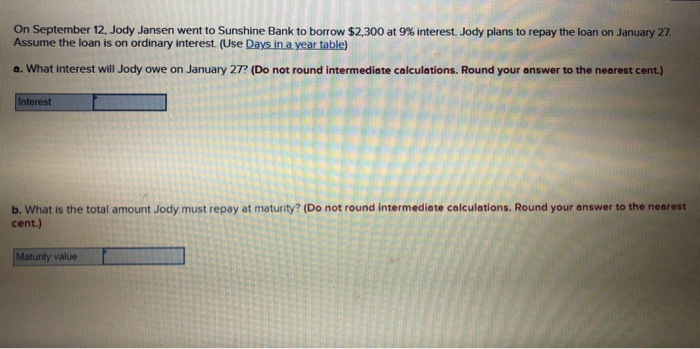On September 12, Jody Jansen went to Sunshine Bank to borrow \$2,300 at 9% interest. Jody plans to repay the loan on January 27 Assume the loan is on ordinary interest. (Use Days in a year table) a. What interest will Jody owe on January 27. (Do not round intermediate calculations. Round your answer to the nearest cent.) Interest b. What is the total amount Jody must repay at maturity? (Do not round Intermediate calculations. Round your answer to the...

• ### The face value of a simple discount note is \$4,000. The bank discount is calculated at...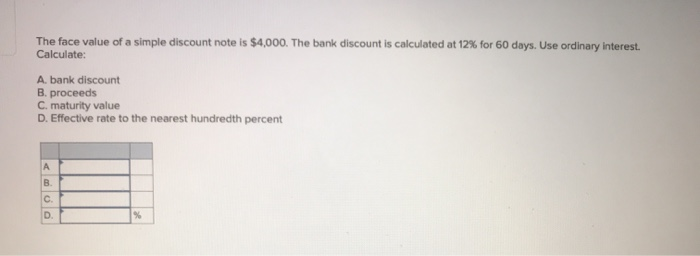The face value of a simple discount note is \$4,000. The bank discount is calculated at 12% for 60 days. Use ordinary interest. Calculate: A bank discount B. proceeds C. maturity value D. Effective rate to the nearest hundredth percent

• ### On May 20, Sheffield Company discounted at Sunshine Bank a \$7,290 (maturity value), 139-day note ...

On May 20, Sheffield Company discounted at Sunshine Bank a \$7,290 (maturity value), 139-day note dated Feb. 20. Sunshine’s discount rate was 8%. (Use Days in a year table.) What proceeds did Sheffield Company receive? (Use 360 days a year. Do not round intermediate calculations.) Proceeds received :?

• ### On September 12, Jody Jansen went to Sunshine Bank to borrow \$200 at 6% interest Jody...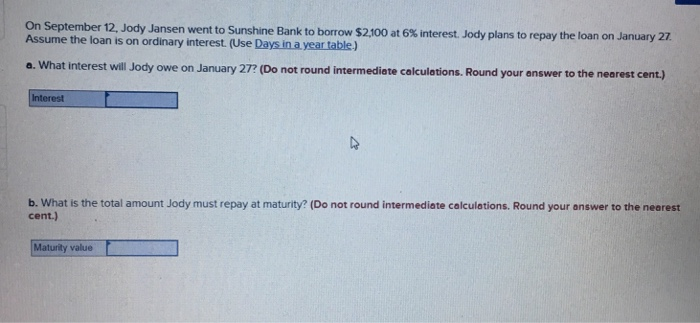On September 12, Jody Jansen went to Sunshine Bank to borrow \$200 at 6% interest Jody plans to repay the loan on January 27 Assume the loan is on ordinary interest. (Use Days in a year table) a. What interest will Jody owe on January 27? (Do not round intermediete calculetions. Round your answer to the nearest cent) b, what is the total amount Jody must repay at maturity? (D。not round intermediate calculations. Round your answer to the nearest cent.)...

• ### On July 14, Jennifer Rick went to Park Bank to borrow \$1,800 at 7 % interest....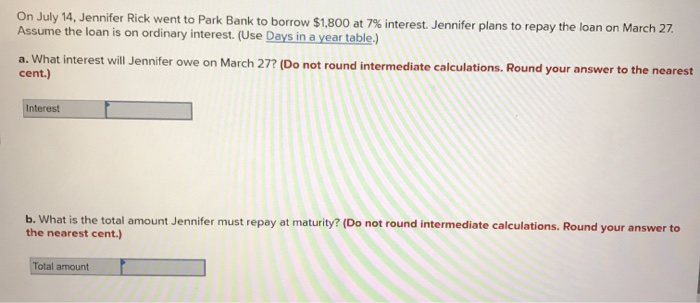On July 14, Jennifer Rick went to Park Bank to borrow \$1,800 at 7 % interest. Jennifer plans to repay the loan on March 27 Assume the loan is on ordinary interest. (Use Days ina year table.) a. What interest will Jennifer owe on March 27? (Do not round intermediate calculations. Round your answer to the nearest cent.) Interest b. What is the total amount Jennifer must repay at maturity? (Do not round intermediate calculations. Round your answer to the...

• ### 8 On October 29, 2019, the Berkeley Company accepted a 60-day, 9 percent note from Devon...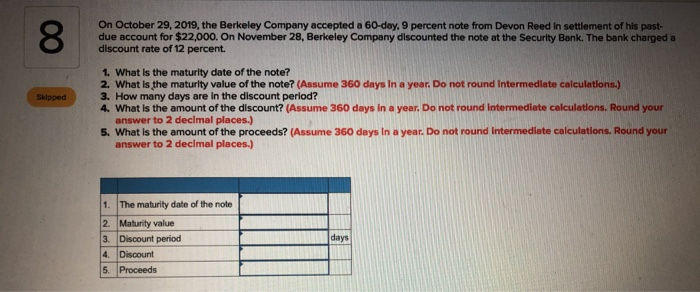8 On October 29, 2019, the Berkeley Company accepted a 60-day, 9 percent note from Devon Reed in settlement of his past- due account for \$22,000. On November 28, Berkeley Company discounted the note at the Security Bank. The bank charged a discount rate of 12 percent. 1. What is the maturity date of the note? 2. What is the maturity value of the note? (Assume 360 days in a year. Do not round Intermediate calculations.) 3. How many days...

• ### Complete the following table for the simple discount notes. Use the ordinary interest method. (Use 360...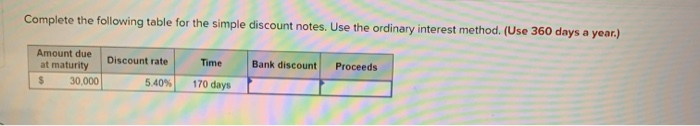Complete the following table for the simple discount notes. Use the ordinary interest method. (Use 360 days a year.) Bank discount Proceeds Amount due at maturity 30.000 Discount rate 5.40% Time 170 days

• ### Please show work :) Complete the following using ordinary interest. (Use Days in a year table.)...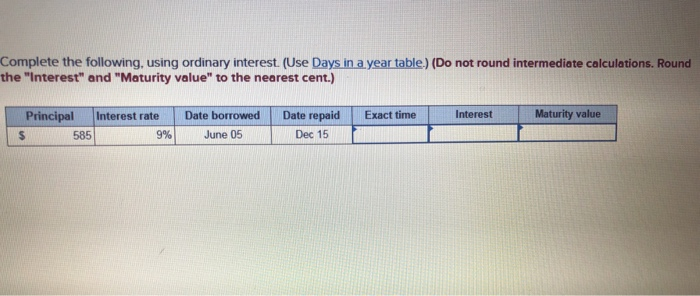Please show work :) Complete the following using ordinary interest. (Use Days in a year table.) (Do not round intermediate calculations. Round the "Interest" and "Maturity value to the nearest cent.) Exact time Interest Maturity value Principal Interest rate \$ 585 9% Date borrowed June 05 Date repaid Dec 15

• ### Given Principal \$14,5ee, Interest Rate 8%, Time 24e days (use ordinary interest) Partial payments: On 108th...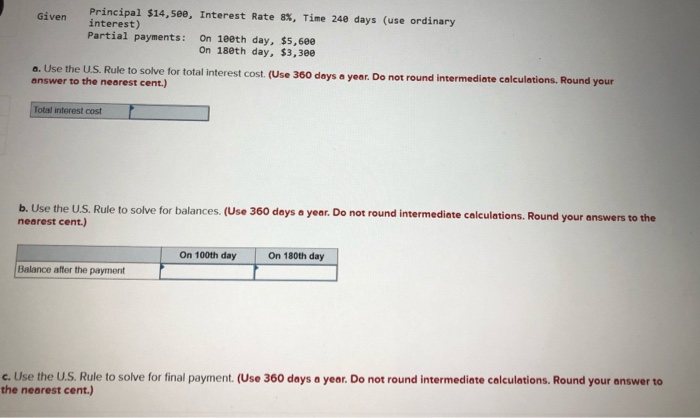Given Principal \$14,5ee, Interest Rate 8%, Time 24e days (use ordinary interest) Partial payments: On 108th day, \$5,6ee On 18eth day, \$3,300 6. Use the U.S. Rule to solve for total interest cost. (Use 360 days a year. Do not round Intermediate calculations. Round your answer to the nearest cent.) Total interest cost | b. Use the U.S. Rule to solve for balances. (Use 360 days a year. Do not round intermediate calculations. Round your answers to the nearest cent.)...